Imagination is more important than knowledge... Albert Einstein

Guess is more important than calculation --- Knowhowacademy.com

Equation - Making equations

For SAT1
Number of Total Problems: 23.
FOR PRINT ::: (Book)

 Problem Num : 1 Type: ????? ??? ???? Section:Equation Theme: Adjustment# : Difficulty: 1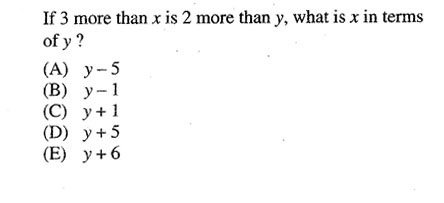Category Making equations
Analysis

 Problem Num : 2 Type: Word problem Section:Equation Theme: Adjustment# : Difficulty: 1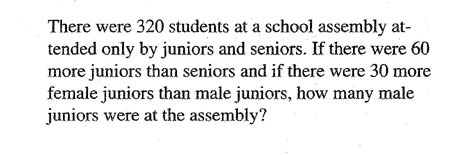Category Making equations
Analysis

 Problem Num : 3 Type: Understanding Section:Equation Theme: Adjustment# : Difficulty: 1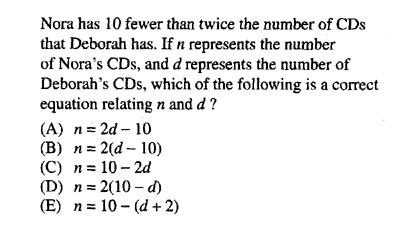Category Making equations
Analysis

 Problem Num : 4 Type: None Section:Equation Theme: Adjustment# : Difficulty: 1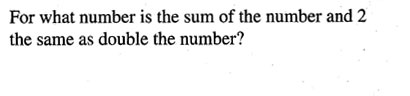Category Making equations
Analysis

 Problem Num : 5 Type: None Section:Equation Theme: Adjustment# : Difficulty: 1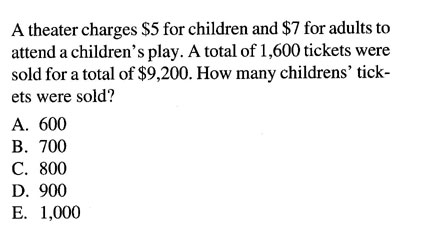Category Making equations
Analysis

 Problem Num : 6 Type: Word problem Section:Equation Theme: Adjustment# : Difficulty: 2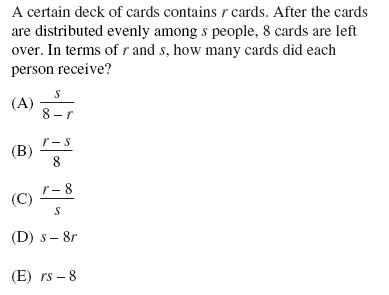Category Making equations
Analysis

 Problem Num : 7 Type: Word problem Section:Equation Theme: Adjustment# : Difficulty: 2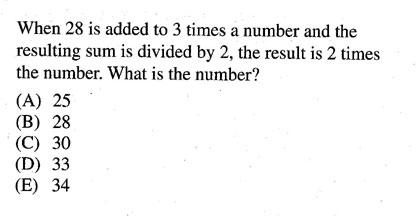Category Making equations
Analysis

 Problem Num : 8 Type: Word problem Section:Equation Theme: Adjustment# : Difficulty: 2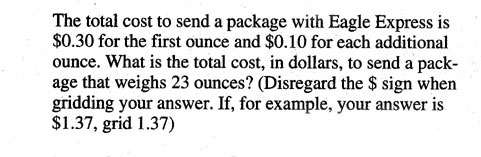Category Making equations
Analysis ??? ?????? ??? ????.

 Problem Num : 9 Type: Many Variables Section:Equation Theme: Adjustment# : Difficulty: 2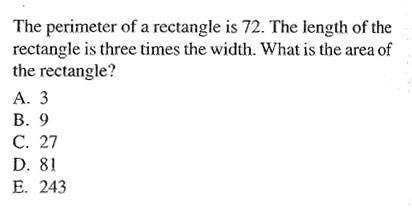Category Making equations
Analysis 2L+2W=72, L=3W

 Problem Num : 10 Type: Many Variables Section:Equation Theme: Adjustment# : Difficulty: 1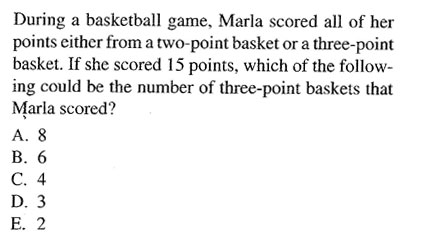Category Making equations
Analysis